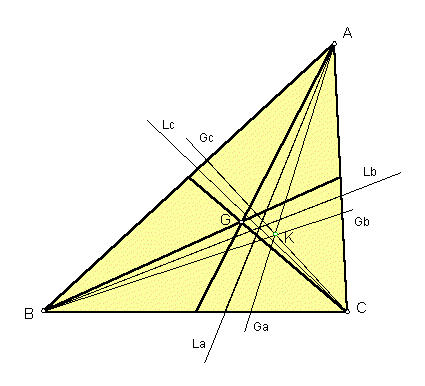# SYMMEDIAN POINT

 In the plane of any triangle ABC, let G be the centroid, and let La = the bisector of angle A, Lb = the bisector of angle B, Lc = the bisector of angle C; Ga = reflection of line AG about La, Gb = reflection of line BG about Lb, Gc = reflection of line CG about Lc. The three lines Ga, Gb, Gc meet in the symmedian point, labeled K in the sketch. It is also known as the Lemoine point. For a particularly good discussion, see Ross Honsberger, Episodes in Nineteenth and Twentieth Century Euclidean Geometry, Mathematical Association of America, Washington, D.C., 1995.Emil Lemoine (1840-1912)
Triangle Centers